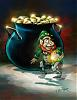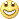# W D Gann Indicators and others - page 8160

If you really want to know how it works you can buy Jim Sloman's ocean book. The movement of the last bar has more influence than what price was 40 bars ago. The natural market river is the summation of the changes in prices over x bars multiplied by the square root of x - (x-1) for each bar back, where x is the number of the bar. We calculate a dynamic ratio at each bar using the natural market river as the numerator and summation of total delta Price calculating the difference in price between each bar. This number is used as a non-arbitrary, dynamically updating, ratio which can be applied to Price as it normally is in an ema, which makes it an nma- natural moving average (because we're using the natural logarithm of price differences) You can also apply the ratio it to the market river itself which makes it a self-adaptive indicator, and when applied to the nmm it bridges the the nmm and nmr, which are based on two totally different calculations. They are in effect feeding off of each other, so when they touch it creates a null zone which indicates a region of price compression followed by a break out. Hope this helps.

update: scalping version for 5M charts.

Sincerely,

Dustin

Files:
nmamm.mq4 15 kb
nmap.gif 23 kb3

dustovshio:
I haven't seen this exact formulas on this forum, nor have I seen them published on the web.

If you want to use a 12 day chart you simply draw a circle place the day you want to use as the last high/low at 0* divide that by 360* and use the following angles 45,60,90,120,135, 180, 225, 240, 270, 315, 330, 360. One whole day is equal to 30* moves. If you use a 24 hr chart each minute is .25*, 5 minutes = 1.25*, 1hr 15*.

In forex you can use either 3,4, or 5 digits in your calculations. For daily, long term go with 5, for intraday use 3. I've attached 3 charts the one with the smaller levels used 3, the other two show 4. Of course it is probably best to use both together to see where they coincide. Use the following formula and chart to calculate price:

1. Decide whether to use 3, 4, or 5 numbers for your price.

2. Take the square root of the last high or low.

!3. Add the equivalent decimal for the angle you want.

4. Square the new number.

45* .25

60* .333

90* .50

120* .666

135* .75

180* 1

225* 1.25

240* 1.333

270* 1.5

300* 1.666

315* 1.75

360* 2

Example (see attached chart) gbp/jpy low 241.97

1. use 4197

2. 64.78

3. 65.78 = 1 + 64.78 or 180*

4. 4327 = 1.4327

On the third chart we used the 4299 low as 0* and find the high of 4431 using 4 number calculation, which was 2 pips from the actual high. The time elapsed was 2hr 50m or 42.5* you can now use 42.5* for the next price calculation as follows:

1. Take the square root of actual high of 4433 = 66.58

2. Subtract the square root of the degrees of time you're interested in. In our case the square root of 42.5 is 6.519 so 66.58 - 6.519 = 60.062.

3. subtract your angles - use 1 for 180* 2 for 360* if you're working with low's add your angles.

4. The first level would be 59.062 * 59.062 (60* this translates to 4 hours time.)

5. New target price is 1.4388 see chart "price-time.

Square of 59.062 is 3488 and NOT 4388 - am I missing something?733

Gann hi lo activator v2 indicator

I have this indicator...what is the logic behind its use?

Any info on it is much appreciated.

Thanks

Files:319

its basically 2 separate 10 period moving averages applied to high and low to make an envelope, when price closes outside this envelope it gives you its signal either buy or sell.733

lepiricus:
its basically 2 separate 10 period moving averages applied to high and low to make an envelope, when price closes outside this envelope it gives you its signal either buy or sell.

But it only draws one line on my chart.319

It draws the line of the side that was breached, if its under your candlesticks it means buy, if it is over your candle stick then sell. Put a 10 period simple moving average on the high and low and take a look, then you'll see how it works298

This indicator was used in the "Auslanco - GBP/JPY and GBP/USD (newstrade)" trading system.298

This indicator is useful too. When the price touch the lower band in the H4 chart, go long. Vice versa for short. I haven't test this technique in other time frame yet. Also check out the time. In this case, I have 87:52 minutes left to go in this H4 bar. If I only have 1 minute left, I shouldn't go long. The next bar could go downhill. In another word, within the 4-hour timeframe, I can go long. After the 4 hour expires, I shouldn't go long. Thanks to Auslanco's tip.

Files:298

I guess we can use this technique in the 30M time frame too. In this case, the price touch the upper band. So we short.

Files:160

mezarashii, here's an example I came across today on the usd/jpy. The spreadsheet and chart are attached. I would have already taken profit because the nmr is going back into the bands. Though I'm simply inquiring if you would think that this would continue to go up based on the information in the time calculator. I have entered the last 4 peaks including the double bottom in fields 1-4.

Regards,

Dustin

Files:
usdjpy1.gif 16 kb
usdjpy30.zip 42 kb